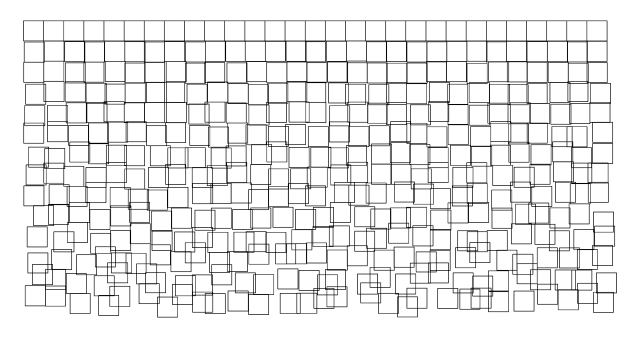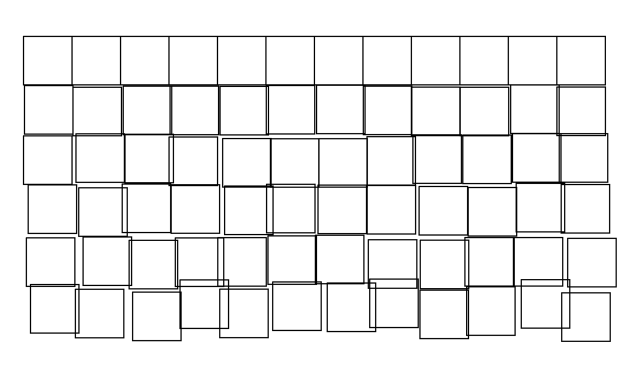## 2019.01.29

### Rectangle ChaosI started playing around with the #plottertwitter thing and wanted to do some creative coding with Processing, and at least one person asked about the code, so I’ve included it below.Instead of drawing a rectangle, I’m creating a shape, and then varying the position of it a bit more with each row that is drawn. I reused some code I’ve used plenty of times before that keeps track of where things are drawn so the code knows when to start a new row, and when the screen is filled. After it draws a screen full it will save a PDF and exit.

Once you have a PDF (which is a vector file) you can make your plotter (or laser cutter, or CNC machine) do what you want with it. I typically bring the PDF into Inkscape and make any adjustments needed before I save it out in a format suitable for the machine I’ll be sending it to.

```/*
* RectangleChaos.pde
*
* Pete Prodoehl <pete@rasterweb.net>
*
* <http://rasterweb.net/raster/>
*
*/

import processing.pdf.*;
import java.util.Date;

int sw = 1280; // if you change the resolution here change
int sh = 720;  // it below in the setup size as well...
Date d = new Date();
long current = d.getTime()/1000;

void setup() {
size(1280, 720);
stroke(0);
strokeWeight(1);
background(255);
beginRecord(PDF, "output-" + current + ".pdf");
}

float xpos = 50;  // you can adjust these numbers to get
float ypos = 50;  // different results, so play around
float wall = 150; // and experiment a bit...
float step = 40;  //
float rsize = 40;
float mimn  = xpos;
float rowct = 0;
float multiple = 0;

void draw() {

noFill();

float x1 = xpos;
float y1 = ypos;

// change number below for different results... try 1.33 or 2.33
multiple = rowct * 2.33;

// first row is normal, after that we offset things a bit
if (rowct > 0) {
x1 = xpos + random(0,multiple);
y1 = ypos + random(0,multiple);
}
else {
x1 = xpos;
y1 = ypos;
}

float x2 = x1 + rsize;
float y2 = y1;

float x3 = x1 + rsize;
float y3 = y1 + rsize;

float x4 = x1;
float y4 = y1 + rsize;

float x5 = x1;
float y5 = y1;

beginShape();
vertex(x1,y1);
vertex(x2,y2);
vertex(x3,y3);
vertex(x4,y4);
vertex(x5,y5);
endShape();

// end of a row
if (xpos > (sw-wall)) {
xpos = mimn;
ypos = ypos + step;
rowct++;
}
else {
xpos = xpos + step;
}

// last row, then we save the PDF and end
if (ypos > (sh-wall)) {
ypos = step;
endRecord();
exit();
}

}
```

If you make something cool with this code, let me know! I’d love to see what you come up with.